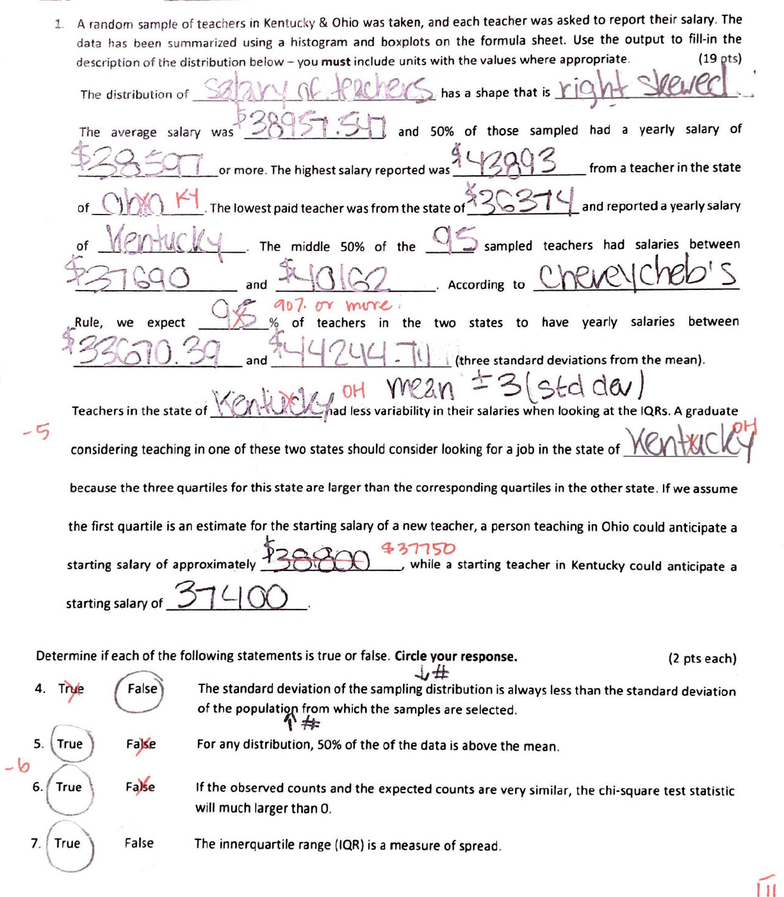Study Guides (390,000)
US (220,000)
NKU (30)
STA (20)
STA 205 (20)
Quiz

# STA 205 Study Guide - Quiz Guide: Quartile, Standard Deviation, Sampling DistributionExam

Department
Statistics
Course Code
STA 205
Professor
Brooke Buckley
Study Guide
Quiz

This preview shows page 1. to view the full 4 pages of the document.1. A random sample
of
teachers in
Ken
t
uc
ky & Ohio
was
taken, and each teacher was asked
to
report
their
salary. The
data
has
been sum
ma
rized usi
ng
a histogram and boxplots on the formula sheet.
Use
the
output
to
fill-in the
description of the distribution below - you must include units
with
the values where appropriate. . ~ 9-i
ts
)
The distdbution of
~h
,
.1__::_1
,
cU12r.Ye.
6 has a shape that
is
ri#
~er.I
-~·
Th
e average
sa
lary wa!
3&
9 S7
;9:D
and
50%
of
those sampled had a yearly salary
of
:t
2a
s;r !
~
lYl
a
fl
~
~:..J
~_ {
_or
more. The highest salary reported was ~
{)
':1
_;
from
a teacher in
the
state
K
of
_C
lOO..J'.:i.
The
lowest paid teacher was
from
the state
of
::1(
3
<:;,
31
<:/
and reported a yearly salary
. The middle
50%
of
the
and \$-\Q\
~2
-9
es
between
.
According
to
C.,'f'RJR\\
dieb
' S
0
~
~
0 7.
(fY
W\,t!Y~
'
)Rule, we expect
__
1
l52.
__
~
of
teachers in the
two
states
to
have yearly salaries between
; 32~ / j .
'?f::/
and ~y Y
Q_
L\LJ
J \ \ , (:three standard deviations
from
the
mean).
Teachers
in
the state
oi
'f
fJo
hltll
va
~:~
t :
a?.1:o
~~
n
~~
e
Ls
considering teaching
in
one
of
these two states should consider
looking
for
a job
in
the
state of
~
C
'l,i
because
the
three quartiles
for
this state are larger than the corresponding quartiles in
the
other
state. If
we
assume
the
first
quartile
is
an estimate
for
the starting salary
of
a new teacher, a person teaching in Ohio could anticipate a
starting salary
of
approximately
\$3e,8:'B
4:1-
~
~~
I
~
starting teacher in Kentucky could anticipate a
starting salary
of
3:t
Lf
00
Determine
if
each
of
the following statements
is
true
or
false.
Circle
your
response.
(2 pts each)
4. T
')(
e
~
The standard deviation
of
the
samplint
!ribution
is
always less than
the
standard deviation
s.8
-lo ~
IIP
\
6
.u
1(
:)
C7
of
the
populati'
tm
which the samples are selected.
False
For any distribution, 50%
of
the
of
the data
is
above the mean.
If
the
observed counts and the expected counts are very similar,
the
chi-square test statistic
will much larger than 0.
The innerquartile range (IQR)
is
a measure
of